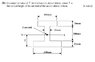# Centre of Area for a I-Section

• thomas55
In summary, the problem is to calculate the value of Y-Bar for the given I-section by finding the centroid of the section above its base. The equation used is Y-Bar x Area of shape = XA * AA + XB * AB + XC * AC, and the final result is Y-Bar = (XA * 3600 + XB * 3600 + XC * 4800) / 12,000. The solution involves finding the centroid Y co-ordinates of the different shapes and using the formula YCM = (m1y1 + m2y2 + m3y3)/ (m1 + m2 + m3). The final answer is 100mm.

#### thomas55

Ok I am completely stuck on this question, i have some idea on how to do it and know it is to do with moments but i can't figure out where to take the moments.

## Homework Statement

Calculate the value of Y-Bar for the I-section shown below, where Y-Bar is the vertical height of the centroid of the section above its base.

## Homework Equations

Y-Bar x Area of shape = XA * AA + XB * AB + XC * AC

## The Attempt at a Solution

Y-Bar x Area of shape = XA * AA + XB * AB + XC * AC

so

Y-Bar x 12,000 = XA * 3600 + XB * 3600 + XC * 4800

Y-Bar = (XA * 3600 + XB * 3600 + XC * 4800) / 12,000

I have gone through my notes and several textbooks but i can't work out where to take the moments to get the XA, XB and XC values. Its probably obvious but if someone can give me a nudge in the right direction it would be appreciated.

#### Attachments

•cog.jpg
16.8 KB · Views: 347

As you need only Y so no need to worry about X(but it will be on the line through the center)

As you are not given anything about mass, i guess you can replace it with area (kind of predictable in most cases as more area means more mass)

Take Y axis passing through the center of body and take origin at center of lower rectangle!

find center of mass Y coordinates of rest 2 rectangles (lower one has (0,0)

use YCM = (m1y1 + m2y2 + m3y3)/ (m1 + m2 + m3)

Im still kinda lost but i have a answer that looks plausible

YCM = (m1y1 + m2y2 + m3y3)/ (m1 + m2 + m3)

YCM = 3600 x 1 + 3600 x 100 + 4800 x 210) / (3600 + 3600 + 4800)

YCM = 114.3mm

Im not sure if i have the y values right though.

thomas55 said:
Im still kinda lost but i have a answer that looks plausible

YCM = (m1y1 + m2y2 + m3y3)/ (m1 + m2 + m3)

YCM = 3600 x 1 + 3600 x 100 + 4800 x 210) / (3600 + 3600 + 4800)

YCM = 114.3mm

Im not sure if i have the y values right though.

Its 0 not 1!
how is Y of this block 210?

My train of thought might be wrong but here goes:

I meant 10 instead of 1 as that would be the centroid Y co-ordinates of the bottom shape

The centroid Y co-ordinates of the top shape is 10 but if you take into account the rest of the shape its 10 + 200 = 210

fixing my calculations i now get 97mm

Edit:

It seems i was taking it for the wrong shape.

Lets try again (I think this is right):

YCM = (10 * 4800 + 110 * 3600 + 210 * 3600) / (4800 + 3600 + 4800)
YCM = 100mm

Last edited:
That looks correct. Note that you summed moment areas about the bottom axis, which is the most convenient way to do this, but you could have summed moments about any horizontal axis, as like the axis cupid.callin suggested, and achieve the same result, but be sure to note that y_bar is measured from the axis about which you are taking moments. Note that this is the area centroid location, not the mass center of mass, which may or may not be equal.

Yes its correct! congratulation!